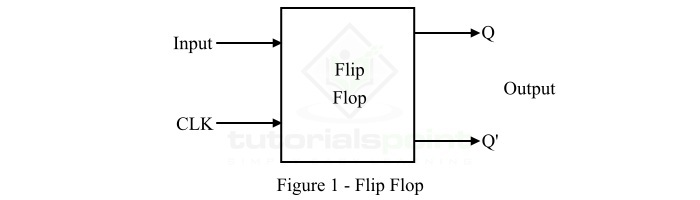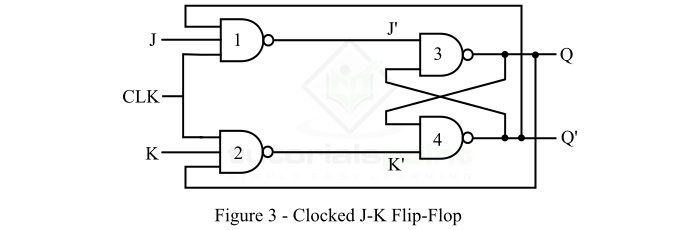# Clocked JK Flip-Flop

In digital electronics, a flip-flop is a most fundamental memory element used in several electronic circuit to store 1-bit information. A flip-flop is a basically a bistable multivibrator having two stable states.

Flip-flops are made up of a combination of logic gates. However, a logic gate itself cannot store information, but when several logic gates are connected in a specific manner, they can store information. Also, flip-flop is the most elementary building block of all sequential logic circuits. The block diagram representation of a flip-flop is shown in Figure-1.A flip-flop has one or more inputs and two outputs, usually represented by Q and Q' along with a clock input. The clock input is used to trigger the flip-flop so that it can change states of its outputs.

There are several types of flip-flops such as S-R flip-flop, J-K flip-flop, D flip-flop, and T flipflop. Each type of flip-flop has its unique properties and characteristics needed for a particular purpose.

This is article is meant for explaining the circuit diagram, truth table, and Boolean expression of the clocked JK flip-flop. So let us start with the basic introduction of the clocked JK flip-flop.

## What is a Clocked JK Flip-Flop?

A flip-flop which has two inputs specified by the two letter J and K respectively is called a JK flip-flop. In the case of JK flip-flip, the symbols J and K are alike to the letter S and R in a SR flip-flop.

Technically, the JK flip-flop is basically a refinement of the SR flip-flop in which the invalid or forbidden state of the SR flip-flop is defined.

The block diagram representation of a JK flip-flop is shown in Figure-2 below.The logic circuit diagram of the JK flip-flop is shown in Figure-3.Hence, the JK flip-flop has two inputs labelled as J and K, and two outputs, Q and Q'. It also has an extra input terminal for clock signal. The clock signal is used to synchronize the flip-flop circuit.

The JK flip-flop is named "JK" in the honor of an inventor Jack Kilby who invented the integrated circuit (IC) in 1958.

## Operation of Clocked JK Flip-Flop

The operation of the above circuit of clocked JK flip-flop is explained below −

When the clock signal is absent, the circuit will remain inactive and the outputs of NAND gates 3 and 4 do not change with any change in the inputs J and K.

When the clock signal is applied to the circuit, the outputs of the NAND gates 3 and 4 will be as per the inputs J and K. In this case, the circuit will operate as described in the following truth table −

InputsPrevious StateOutput(Next State)Comment
JKQnQn+1
0000No Change
0011No Change
0100Reset
0110Reset
1001Set
1011Set
1101Toggle
1110Toggle

From this truth table of the clocked JK flip-flop, we can derive the characteristic equation of the flip-flop as follows −Hence, the characteristic equation of the JK flip-flop is,

$$Q_{n+1}=JQ_{n}^{'}+K'Q_{n}$$

## Advantages of Clocked JK Flip-flop

The following are the major advantages of JK flip-flop −

• In JK flip-flop, the forbidden state does not occur.

• In JK flip-flop, instead of forbidden state, the present state toggles, i.e. the present state gets complemented when both the inputs (J and K) are 1.

This is all about the clocked JK flip-flop in digital electronics.

Updated on: 25-Apr-2023

945 Views